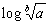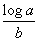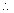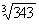## How to Use Logarithms (cont.)

 whose mantissa is not exactly given in the table Subtraction of logarithms 1. Find the tabular difference between the next highest and next lowest man- tissa. 4.1076.986 = 14.107 - 106.9867.121 - 10 2. Divide this by the differnece between the given mantissa and the next low- est mantissa. 11.672 5.7855.887 - 10- 10 3. Add the resulting quotient to the significant figures expressed by the next lower mantissa. The relationships of logarithmic opera- tions are expressed by the following formu- las: 4. Place the decimal as indicated by the given characteristic. log (a * b) log ( a / b ) == log a + log b log a - log b Example: Find the antilog of 1.7376 log (ab) = b log a Next higher mantissa Next lower mantissa 0.73800.7372=Tabular difference 0.0008 EXAMPLES Given mantissa Next lower mantissa 0.73760.7372 To Multiply               961 log of 1.24 by= 2240.0934 Tabular difference 0.0004 0.0004 log of  246 Total = 2.39090.6325 Quotient of 0.0008 = 0.5000 The antilog of 2.4843 = 305, which is as The resultant figure therefore is 0.5 larger than the significant figures expressed by the lesser mantissa 0.7372 or 546. The sequence accurate as can be determined with a four- place table. The full answer to this prob- lem is 305.04. of figures therefore is 546.5. To Divide                  961 by 224the antilog of 1.7376 = 54.65 log of 961 = 2.9827 log of 224 = 2.3502 Note: When interpolating as shown above, do not exceed four significant figures in your answer since interpolated results from a four-place table are not accurate beyond this point.    Logarithms are added or subtracted like arithmetical numbers, provided they are written with positive characteristics. If the characteristic in the total is greater than 9, and the notation -10, -20, -30, etc., appears after the mantissa, subtract a mul- tiple of 10 from the positive part and add the same multiple of 10 to the negative part, so as to make the resultant character- istic less than 10. Difference 0.6325 The antilog of 0.6325 = 4.29 which is as accurate as can be determined with a four- place table table. The product of 224 and 4.29 is 960.96 Powers: Find 122 by logarithms: log of 12 = 1.0792     *  22.1584 The antilog of 2.158 = 144. Roots                    Findlog of 343 = 2.5353 / 3 The antilog of 0.8451 == 0.84517.00 EXAMPLES: Logarithms of Negative Numbers. Be- cause the logarithms of negative numbers are imaginary in character, they cannot be used in computation as with positive num- bers. However, since the numerical results of multiplying, dividing, etc., are not affected by the signs, you can determine the numerical results by logarithms and later affix the final + or - signs by inspection. Addition of logarithms 2.7644.3047.068 6.326 - 10  6.284         12.610 - 10or2.610 6.328 - 10  7.764 - 10   9.104 - 1023.196 - 30 or3.196 - 10